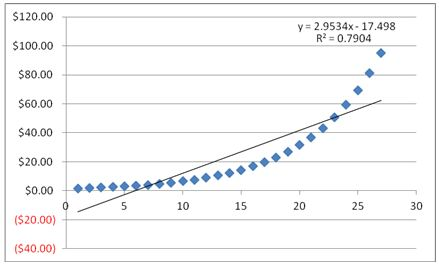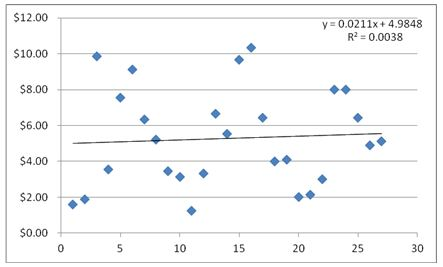# Regression

Updated November 4, 2020

## What is Regression?

Regression is a statistical method used in finance and other fields to make predictions based on observed values. It is a measure of how correlated a group of actual observations are to a model’s predictions.

## How Does Regression Work?

In the following examples, the blue dots represent the going prices for figurine collections on eBay. Collections with more figurines go for as much as \$100; collections with fewer than five figurines sell for very little. How can we predict how much a collection will sell for?

We do it using regression analysis, which essentially finds the formula for the line that most closely fits the observations. That way, we can use the line to predict what the price of the collection might be if we know how many figurines are in a collection, or we can predict how many figurines should be in a collection if we know the asking price.

In our example below, the black lines represent a regression line, which is represented by the formula in the top right-hand corner of each chart. This formula is what analysts also use to predict future values of securities based on the behavior of the actual observations.

Goodness of fit is a component of regression analysis. The term refers to how far apart the expected values of a financial model are from the actual values (that is, how predictive the line is).

As you can see, this regression line has a high goodness of fit; the formula for the regression line comes up with the observed values about 79% of the time.This next chart is an example of a regression line with low goodness of fit. Here, the values are all over the place, and the formula for the regression line was virtually unable to predict anything.## Why Does Regression Matter?

Regression is a mathematical version of a crystal ball, but a very cracked, blurry crystal ball. Goodness of fit is the key -- it's a confidence measure. This is because when you've come up with a formula that accounts for most of the variations in a group of, say, price observations, you've also come up with a formula that can be a very reliable predictor of what prices will be in the future. And that’s priceless.

All of our content is verified for accuracy by Paul Tracy and our team of certified financial experts. We pride ourselves on quality, research, and transparency, and we value your feedback. Below you'll find answers to some of the most common reader questions about Regression.
##### Be the first to ask a question

Paul Tracy - 140Paul has been a respected figure in the financial markets for more than two decades. Prior to starting InvestingAnswers, Paul founded and managed one of the most influential investment research firms in America, with more than 2 million monthly readers.